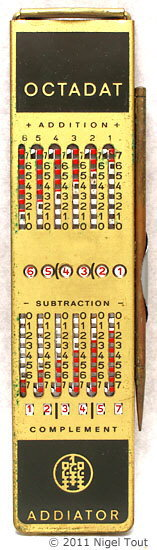Non-Decimal Calculators

Home > Non-Decimal Calculators > Time Calculators

Calculators able to work in the Octal and Hexadecimal number systems are very useful to computer programmers since these are useful ways in representing the binary numbers used by computers.

The calculators shown here are:

• Texas Instruments Programmer - Hand-held electronic calculator capable of working in the octal and hexadecimal systems.This is an "Addiator"-type stylus calculator using the octal number system.

42 x 160 x 4 mm (1.7" x 6.3" x 0.2").

The octal number system uses numbers to the base 8, from 1 to 7.
So the photograph shows the octal number 654321, which represents the binary number 110 101 100 011 010 001, or 219,345 in the decimal system.
The octal system is largely obsolete now but was widely used with earlier electronic computers.

Was available from Radix Precision, Atlanta GA, U.S.A. for US\$14.95 and from Data Efficiency, UK.Hexadat calculator with its stylus, which has a propelling pencil (mechanical pencil) at the other end, which would be useful for writing results.

61 x 232 x 4 mm (2.4" x 9.1" x 0.2").

The hexadecimal number system uses numbers to the base 16, from 1 to 15 written using the numbers 1 to 9 and continuing with the letters A to F.
So the photographs show the hexadecimal number 1ECA8642, which represents the binary number 0001 1110 1100 1010 1000 0110 0100 0010, or 516,589,122 in the decimal system.

The hexadecimal system is widely used when programming with electronic computers.

As shown in the photograph in the hand, this model is much larger than the normal small Addiators.

The journal 'Electronics Design' announced a "Programmer's Kit" in 1971 which contained several programming aids:
"Developed to assist programmers is a new kit of ten handy calculating tools.  It contains a small machine known as the Hexadat which calculates hexadecimal values composed of numbers and letters, a universal decimal calculator and a conversion table.  In addition, the kit contains a slide rule, a programmer's stencil and drawing pencils and refills.  The entire set comes in a black leather briefcase.

The Hexadat was available on its own in 1976 for US\$35.95 from Radix Precision, Atlanta GA, U.S.A.Texas Instruments ProgrammerTexas Instrument Programmer

This is a standard 4-function hand-held electronic calculator which is able to operate in the decimal, octal, and hexadecimal number systems. As well as being able to perform the four arithmetical functions this calculator can also perform logical operations and to convert a number between the different number systems.

The photograph shows the calculator displaying the hexadecimal number ABCDEF12, which represents the binary number 1010 1011 1100 1101 1110 1111 0001 0010, or 2,882,400,018 in the decimal system.

The hexadecimal system is widely used when programming electronic computers, and so the successors to this model are still being used.

Reference:

1. "What's New", Byte, July 1976, p102 & p112.
2. Data Processing, March-April 1971, p120.
3. "Programmer's kit", Electronic Design, September 1971, p88.

Non-decimal calculators

Vintage Calculators

© Text & photographs copyright Nigel Tout   2000-2022  except where noted otherwise.